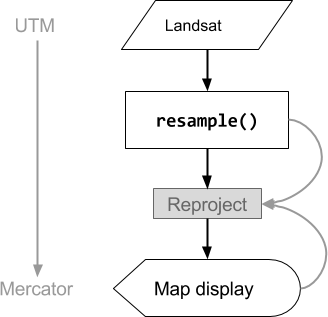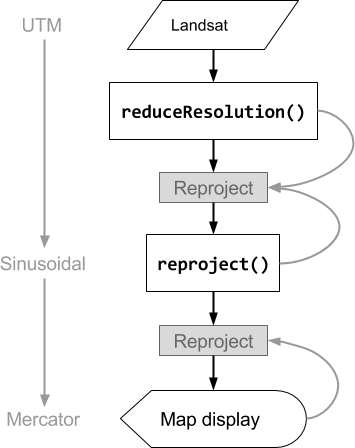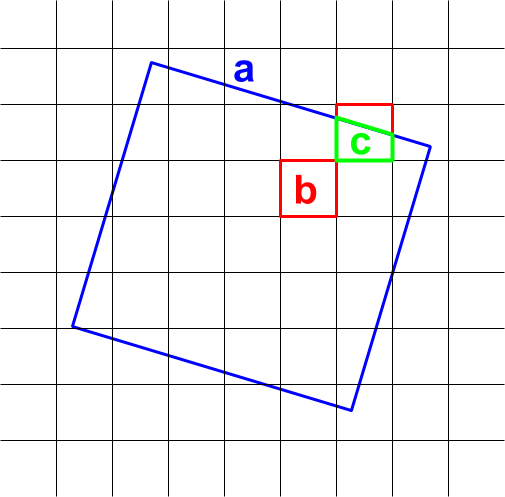Resampling and Reducing Resolution

As noted in the Projections doc, Earth Engine performs nearest neighbor resampling by default during reprojection. You can change this behavior with the resample() or reduceResolution() methods. Specifically, when one of these methods is applied to an input image, any required reprojection of the input will be done using the indicated resampling or aggregation method.

Resampling

resample() causes the indicated resampling method ('bilinear' or 'bicubic') to be used at the next reprojection. Since inputs are requested in the output projection, an implicit reprojection may happen before any other operation on the input. For this reason, call resample() directly on the input image. Consider the following simple example:

// Load a Landsat image over San Francisco, California, UAS.
var landsat = ee.Image('LANDSAT/LC08/C01/T1_TOA/LC08_044034_20160323');

// Set display and visualization parameters.
Map.setCenter(-122.37383, 37.6193, 15);
var visParams = {bands: ['B4', 'B3', 'B2'], max: 0.3};

// Display the Landsat image using the default nearest neighbor resampling.
// when reprojecting to Mercator for the Code Editor map.
Map.addLayer(landsat, visParams, 'original image');

// Force the next reprojection on this image to use bicubic resampling.
var resampled = landsat.resample('bicubic');

// Display the Landsat image using bicubic resampling.

Note that the 'bicubic' resampling results in the output pixels appearing smooth relative to the original image (Figure 1).

The order of operations for this code sample is diagrammed in Figure 2. Specifically, the implicit reprojection to the maps mercator projection takes place with the resampling method specified on the input image.Figure 2. Flow chart of operations when resample() is called on the input image prior to display in the Code Editor. Curved lines indicate the flow of information to the reprojection: specifically, the output projection, scale and resampling method to use.

Reduce Resolution

Suppose that instead of resampling during reprojection, your goal is to aggregate pixels to larger pixels in a different projection. This is useful when comparing image datasets at different scales, for example 30-meter pixels from a Landsat-based product to coarse pixels (higher scale) from a MODIS-based product. You can control this aggregation process with the reduceResolution() method. As with resample(), call reduceResolution() on the input, in order to affect the next reprojection of the image. The following example uses reduceResolution() to compare forest cover data at 30-meters resolution to a vegetation index at 500-meters resolution:

// Load a MODIS EVI image.
var modis = ee.Image(ee.ImageCollection('MODIS/006/MOD13A1').first())
.select('EVI');

// Display the EVI image near La Honda, California.
Map.setCenter(-122.3616, 37.5331, 12);
Map.addLayer(modis, {min: 2000, max: 5000}, 'MODIS EVI');

// Get information about the MODIS projection.
var modisProjection = modis.projection();
print('MODIS projection:', modisProjection);

// Load and display forest cover data at 30 meters resolution.
var forest = ee.Image('UMD/hansen/global_forest_change_2015')
.select('treecover2000');
Map.addLayer(forest, {max: 80}, 'forest cover 30 m');

// Get the forest cover data at MODIS scale and projection.
var forestMean = forest
// Force the next reprojection to aggregate instead of resampling.
.reduceResolution({
reducer: ee.Reducer.mean(),
maxPixels: 1024
})
// Request the data at the scale and projection of the MODIS image.
.reproject({
crs: modisProjection
});

// Display the aggregated, reprojected forest cover data.
Map.addLayer(forestMean, {max: 80}, 'forest cover at MODIS scale');

In this example, note that the output projection is explicitly set with reproject(). During the reprojection to the MODIS sinusoidal projection, rather than resampling, the smaller pixels are aggregated with the specified reducer (ee.Reducer.mean() in the example). This sequence of operations is illustrated in Figure 3. Although this example uses reproject() to help visualize the effect of reduceResolution(), most scripts don't need to explicitly reproject; see the warning here.Figure 3. Flow chart of operations when reduceResolution() is called on an input image prior to reproject(). Curved lines indicate the flow of information to the reprojection: specifically, the output projection, scale and pixel aggregation method to use.

Note that a second reprojection occurs (implicitly) to display the data on the Code Editor map. Visually inspect the results and observe the correspondence between the pixels from the MODIS layer and the forest cover data reprojected to MODIS scale and projection. In general, you should rarely need to explicitly reproject() in Earth Engine.

Pixel weights for ReduceResolution

The weights of pixels used during the reduceResolution() aggregation process are based on the overlap between the smaller pixels being aggregated and the larger pixels specified by the output projection. This is illustrated in Figure 4.Figure 4. Input pixels (black) and output pixel (blue) for reduceResolution().

The default behavior is that input pixel weights are computed as the fraction of the output pixel area covered by the input pixel. In the diagram, the output pixel has area a, the weight of the input pixel with intersection area b is computed as b/a and the weight of the input pixel with intersection area c is computed as c/a. This behavior can result in unexpected results when using a reducer other than the mean reducer. For example, to compute forested area per pixel, use the mean reducer to compute the fraction of a pixel covered, then multiply by area (instead of computing areas in the smaller pixels then adding them up with the sum reducer):

// Compute forest area per MODIS pixel.
var forestArea = forest.gt(0)
// Force the next reprojection to aggregate instead of resampling.
.reduceResolution({
reducer: ee.Reducer.mean(),
maxPixels: 1024
})
// The reduce resolution returns the fraction of the MODIS pixel
// that's covered by 30 meter forest pixels.  Convert to area
// after the reduceResolution() call.
.multiply(ee.Image.pixelArea())
// Request the data at the scale and projection of the MODIS image.
.reproject({
crs: modisProjection
});
Map.addLayer(forestArea, {max: 500 * 500}, 'forested area at MODIS scale');